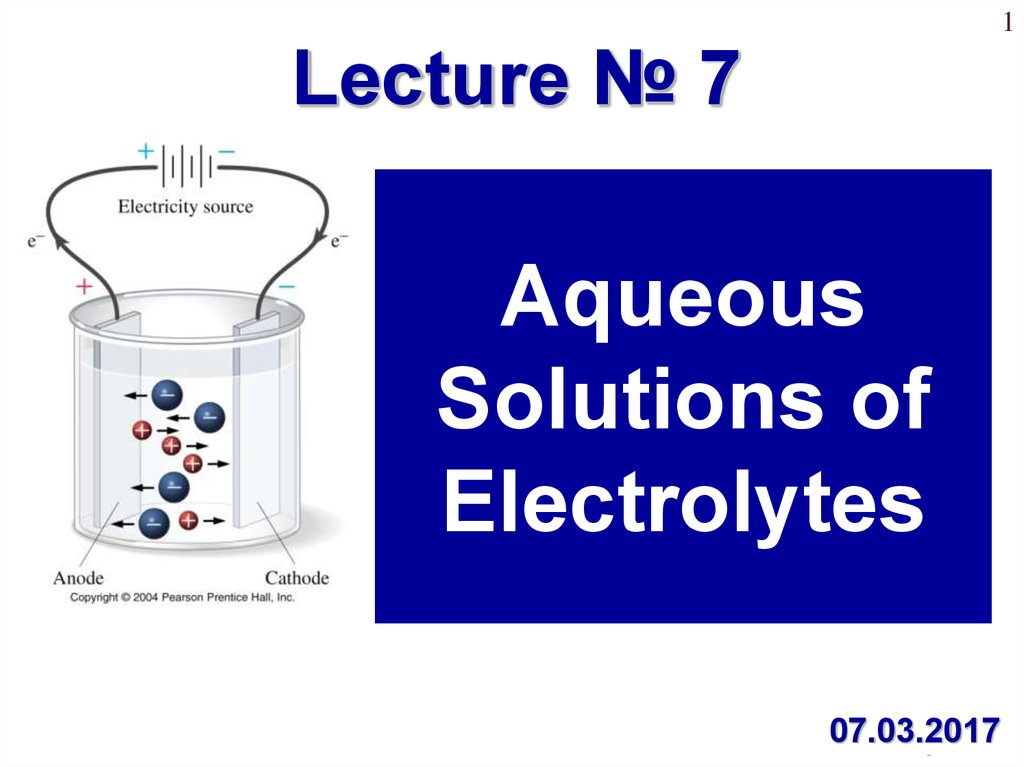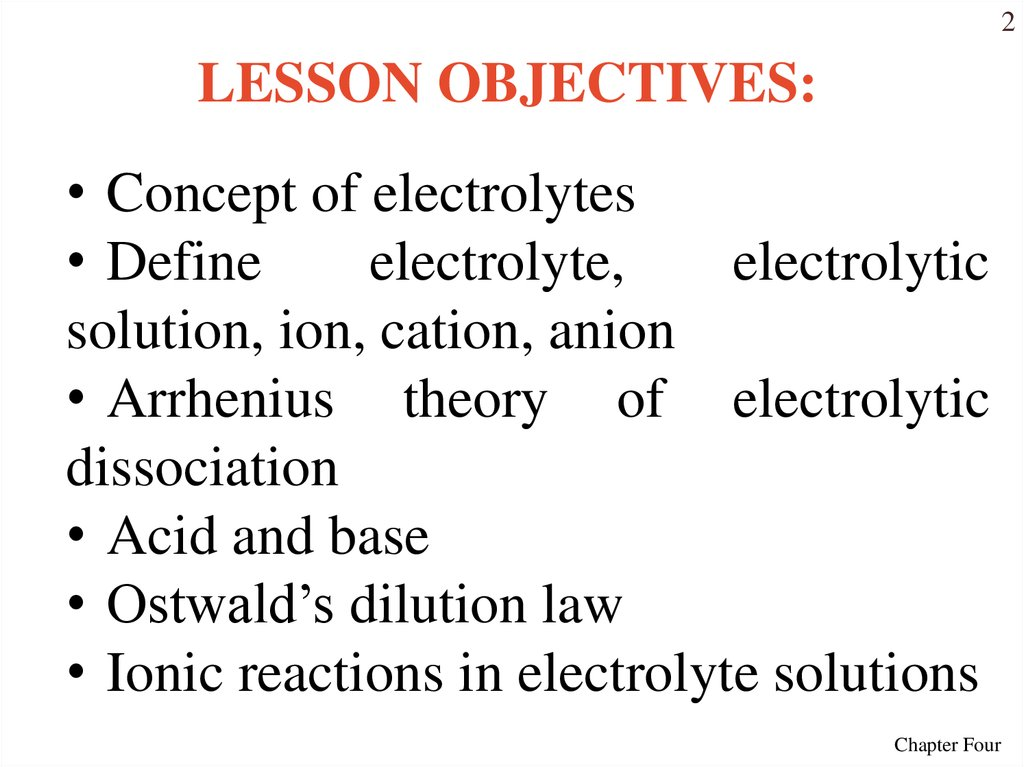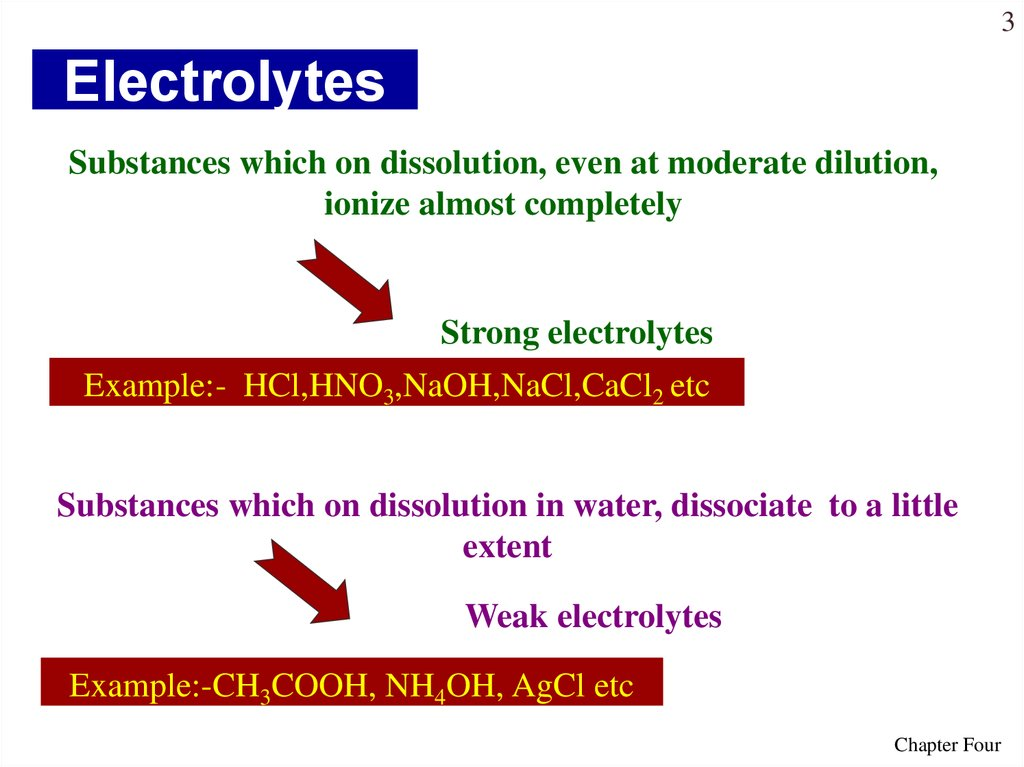# Aqueous Solutions of Electrolytes

1
Lecture № 7
Aqueous
Solutions of
Electrolytes
07.03.2017
Chapter Four

## 2.

2
LESSON OBJECTIVES:
• Concept of electrolytes
• Define
electrolyte,
electrolytic
solution, ion, cation, anion
• Arrhenius theory of electrolytic
dissociation
• Acid and base
• Ostwald’s dilution law
• Ionic reactions in electrolyte solutions
Chapter Four

## 3. Electrolytes

3
Electrolytes
Substances which on dissolution, even at moderate dilution,
ionize almost completely
Strong electrolytes
Example:- HCl,HNO3,NaOH,NaCl,CaCl2 etc
Substances which on dissolution in water, dissociate to a little
extent
Weak electrolytes
Example:-CH3COOH, NH4OH, AgCl etc
Chapter Four

## 4.

4
In the world of chemistry, an electrolyte is a
substance having the free ions so that the
substance is electrical conductor. We can say
that any substance, which furnishes ions in
the solution, is called the electrolyte.
Due to the presence of free ions some of the
solutions can pass electricity through them. As we
can say that the pure distilled water is not an
electrolyte but if we add some table salt, it
becomes an electrolyte and the electric current
pass through it.
The electrolyte is an ionic solution but
the electrolytes can also be in molten and in
the solid state.
Chapter Four

## 5. Pure water does not conduct an electric current

5
Pure water does
not conduct an
electric current
Source of
electric power
Source of
electric power
Pure
water
Zumdahl, Zumdahl, DeCoste, World of Chemistry 2002, page 215
Ionic Solutions
conduct a
current
Free ions
present
in water
Chapter Four

## 6. Electrostatic Forces

6
Electrostatic Forces
• Unlike charges (+ and –) attract one another.
• Like charges (+ and +, or – and –) repel one another.
Chapter Four

## 7.

7
Electric current is a movement or flow
of electrically charged particles (electrons
and ions), typically measured in:
Q I t
Charged particles capable of conducting
electrical current are called electrical
conductors:
• Metals are conductors of the first type
with an electronic conductivity
• Electrolytes are conductors of the
second type with an ionic conductivity
Chapter Four

## 8.

8
Cathode
cations
Anode
anions
Ions are atoms (or groups of atoms) that
carry electrical charge.
Positively (+) charged ions are called
cations, whereas negatively (–) charged ions
are called anions.
Chapter Four

## 9.

• Electrolytes are
substances that
dissociate into
ions when
dissolved in water
and conduct
electricity
• Non-electrolytes
are
substances
that do not form
ions and do not
conduct
electricity when
placed in water
Chapter Four
9

## 10.

Electrical Conductivity of Ionic Solutions
10
Chapter Four

## 11.

11
The more the electrolyte dissociates, the more ions it
produces.
Chapter Four

## 12.

12
TYPES OF ELECTROLYTES
A weak electrolyte
dissociates partially.
• Weak
electrolyte
solutions
are
poor
conductors.
• Different
weak
electrolytes dissociate to
different extents.
Weak electrolytes
include:
• Weak acids and weak
bases (NH4OH)
• A few insoluble ionic
compounds
• A water H2O
A strong electrolyte
dissociates completely.
• A strong electrolyte is
present in solution almost
exclusively as ions.
• Strong
electrolyte
solutions
are
good
conductors.
Strong electrolytes
include:
• Strong acids (HCl, HBr, HI,
HNO3, H2SO4, HClO4)
• Strong bases (IA and IIA
metals hydroxides)
• Most water-soluble ionic
compounds (salts)
Chapter Four

13
dipol
Chapter Four

## 14.

14
Water is “bent” or V-shaped.
The O – H bonds are covalent.
Water is a polar molecule.
Hydration occurs when salts dissolve in water.
Chapter Four

## 15. How Water Dissolves an Ionic Substance

15
How Water Dissolves an Ionic Substance
H2O
hydrated anion
Cl
Na
hydrated cation
Chapter Four

16
Chapter Four

## 17.

Теория электролитической диссоциации
17
Этапы электролитической диссоциации полярных молекул (а) и
ионных кристаллов (б): I – сольватация; II – ионизация; III –
Chapter Four
диссоциация.

## 18.

18
ARRHENIUS THEORY OF
ELECTROLYTIC
DISSOCIATION
In order to explain the
properties
of
electrolytic
solutions, Arrhenius put forth,
in 1884, a comprehensive
theory which is known
as theory of electrolytic
dissociation or ionic theory.
Svante Arrhenius,
Swedish chemist
and
Nobel laureate,
1859-1927.
Chapter Four

## 19.

THE MAIN POINTS OF THE THEORY ARE:
19
• An electrolyte, when dissolved in water, breaks
up into two types of charged particles. These
charged particles are called ions.
• When an electric current is passed through the
electrolytic solution, the positive ions (cations)
move towards cathode and the negative ions
(anions) move towards anode and get discharged,
i.e., electrolysis occurs.
• The process of splitting of the electrolytes
molecules into ions under the influence of polar
molecules of solvent is called ionization
m
n
(dissociation):
K x Ay xK
cation
yA
Chapter Four
anion

## 20.

20
• The properties of electrolytes in solution are
the properties of ions present in solution. For
example, acidic solution always contains H+ ions
while basic solution contains OH- ions and
characteristic properties of solutions are those of Hions and OH- ions respectively.
• The ions act like molecules towards depressing the
freezing point, elevating the boiling point, lowering the
vapour pressure and establishing the osmotic
pressure.
• The conductively of the electrolytic solution
depends on the nature and number of ions as the
current is carried through solution by the movement
of ions.
Chapter Four

## 21.

21
An acid is a substance that
increase H+ when dissolved in water:
HNO3 ( aq) H
( aq)
3( aq )
NO
Some acids have more than one
ionizable hydrogen atom. They ionize in
“steps”:
H2SO4 → H+ + HSO4–
hydrogen sulfate ion
HSO4– → H+ + SO42–
sulfate ion
Chapter Four

## 22. Reactions of Acid: Strong and Weak Acids

22
Reactions of Acid:
Strong and Weak Acids
• Strong acids are strong electrolytes; completely
ionized in water:
In water:
HCl(g) → H+(aq) + Cl–(aq)
No HCl in
solution, only H+
and Cl– ions.
• Weak acids are weak electrolytes. Some of the
dissolved molecules ionize; the rest remain as
molecules.
In water: CH3COOH(l) H+(aq) + CH3COO–(aq)
Just a little H+ forms.
Chapter Four

## 23.

23
A base is a substance that
increase OH- when dissolved in water:
NaOH ( aq) Na
( aq)
OH
( aq)
An ampholyte is a substance
dissolving in water to OH- and H+ ions:
H 2 O H OH
Zn(OH ) 2 Zn
2
2OH
H 2 ZnO2 2 H ZnO
2
2
Chapter Four

## 24. Strong and Weak Bases

24
Strong and Weak Bases
• Strong bases: Most are ionic hydroxides (Group IA and IIA,
though some IIA hydroxides aren’t very soluble).
• Weak bases: Like weak acids, they ionize partially.
Ionization process is different.
• Weak bases form OH– by accepting H+ from water …
NH3 + H2O
CH3NH2 + H2O
methylamine
Most of the weak base remains
in the molecular form.
NH4+ + OH–
CH3NH3+
OH–
+
methylammonium ion
Just a little OH– forms.
Chapter Four

## 25. Common Strong Acids and Strong Bases

25
Common Strong Acids
and Strong Bases
A pragmatic method of determining
whether an acid is weak … just learn
the strong acids!
Chapter Four

## 26.

26
Strength and properties of the
electrolyte are described by the following
values:
• the degree ( ) of ionization
• and the dissociation constant (Kdiss) of the
electrolyte.
Degree of ionization ' ' may be defined
as a fraction of total number of molecules
of an electrolyte which dissociate into ions:
n (number of molecules dissociate d int o ions )
N (Total number of molecules )
100%
Chapter Four

## 27.

27
Values of the degree of dissociation (ex)depends upon the following
factors
1. nature of solute
2. nature of solvent
3. concentration
4. temperature
Chapter Four

## 28.

28
When temperature
is increased, degree
of ionization
increase too
When concentration
is increased, degree
of ionization
decrease
Chapter Four

## 29.

29
Dissociation is reversible process. Ions
present in solution constantly re-unite to form
neutral molecules and, thus, there is a state of
dynamic equilibrium between the ionized and
non-ionized
molecules,
i.e.,
AxBy x A+ + y B-
Applying the law of mass action to above
equilibrium, we have constant K is known as
x
y
ionization constant :
[ A ] [B ]
K diss
[ Ax B y ]
Chapter Four

## 30.

30
a) H 3 PO4 3H PO43
K дисс
[ H ]3 [ PO43 ]
[ H 3 PO4 ]
b) Cu (OH ) 2 Cu 2 2OH
K дисс
[Cu 2 ] [OH ] 2
[Cu (OH ) 2 ]
• For strong electrolytes >0,3 (30%)
and they having high value of Kdiss
• For weak electrolytes <0,3 (0 –
30%) and those having low constant
value of Kdiss
Chapter Four

## 31.

OSTWALD'S DILUTION LAW
31
• This law is based on the fact that only a portion of the
electrolyte is dissociated into ions at ordinary dilution and
completely at infinite dilution. Strong electrolytes are
almost completely ionized at all dilutions and / ∞ does
not give an accurate value of 'α'.
• When the concentration of ions is very high, the
presence of charges on the ions appreciably affects the
equilibrium. Hence, law of mass action cannot be strictly
applied in the case of strong electrolytes.
• For strong electrolytes:
K diss
2 CM
1
where K is dissociation constant and C is molar concentration of the solution.
Chapter Four

## 32.

OSTWALD'S DILUTION LAW
32
• For weak electrolytes (Kdiss=const
and (1 – ) 1):
K diss С М
2
K diss
CM
Thus, degree of dissociation of a weak electrolyte is
proportional to the square root of dilution.
“For a weak electrolyte, the degree of ionisation
is inversely proportional to the square root of molar
concentration or directly proportional to the square
root of volume containing one mole of the solute.”
This is called Ostwald’s dilution law.
Chapter Four

## 33.

33
IONIC REACTIONS
A chemical equation which shows
dissociation of electrolyte and written
as dissociated ions of electrolyte is
known as ionic equation.
Double Replacement (Metathesis)
Reactions involve swapping ions in
solution:
AX + BY AY + BX
Chapter Four

## 34.

34
Metathesis reactions will lead to a
change in solution if one of three things
occurs:
• An insoluble solid is formed (When a
chemical reaction forms such a solute, the
insoluble solute comes out of solution and is
called a precipitate),
• Weak
acids
are
formed
(H2SO3,
CH3COOH),
• An insoluble gas is formed (H2S, CO2,
SO2, NH3),
• Neutralization reaction
Chapter Four

## 35.

35
IONIC REACTION
Irreversible reaction
Precipitate(↓)
BaCl2 + Na2SO4 = BaSO4 ↓ +
2NaCl
Gas reaction
Na2S+ 2HCl = H2S↑ + 2NaCl
Weak electrolytes are
formed
2CH3COOK + H2SO4 =
= 2CH3COOH + K2SO4
Complex compounds
are formed
Hg(NO3)2 + 4KJ = K2[HgJ4]
+2KNO3
Chapter Four

## 36. Acid–Base Reactions: Neutralization

36
Acid–Base Reactions:
Neutralization
Neutralization is the (usually
complete) reaction of an acid with a
base. The products of this
neutralization are water and a salt.
NaOH ( aq) HCl ( aq) NaCl( aq) H 2 O(l )
Chapter Four

## 37. Acid–Base Reactions: Net Ionic Equations

37
Acid–Base Reactions:
Net Ionic Equations
HCl + NaOH H2O + NaCl
• In the reaction above, the HCl, NaOH, and NaCl
all are strong electrolytes and dissociate
completely.
Na+ and Cl–
are spectator
• The actual reaction occurs between ions.
ions.
H+ + Cl– + Na+ + OH– H2O + Na+ + Cl–
H+ + OH– H2O
A net ionic equation shows the species actually involved in the reaction.
Chapter Four

## 38. Reactions that Form Precipitates

38
Reactions that Form Precipitates
• There are limits to the amount of a solute that
will dissolve in a given amount of water.
• If the maximum concentration of solute is less
than about 0.01 M, we refer to the solute as
insoluble in water.
• When a chemical reaction forms such a solute,
the insoluble solute comes out of solution and is
called a precipitate.
Chapter Four

## 39. Silver Iodide Precipitation

39
Silver Iodide Precipitation
A solution containing
silver ions and nitrate
… a precipitate of
silver iodide.
… a solution
containing potassium
ions and iodide ions,
forms …
What is the net ionic
equation for the
reaction that has
occurred here? (Hint:
what species actually
reacted?)
Chapter Four

## 40.

40
• With these guidelines we can predict precipitation
reactions.
• When solutions of sodium carbonate and iron (III)
nitrate are mixed, a precipitate will form.
• When solutions of lead acetate and calcium chloride
are mixed, a precipitate will form.
Chapter Four

41
Chapter Four